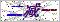订购服务热线： 400-053-8065首页 - 产品中心 - 工业控制传感器/变送器 - 温度传感器/变送器（热电偶、热电阻） - pt100温度传感器
 产品目录工业控制传感器/变送器产品中心点击放大
 pt100温度传感器
 产品型号： 产品报价：产品特点： pt100温度传感器、非标国定法兰式热电偶、热电阻PT100传感器技术参数铂热电阻：（-200～600）℃ 精度： A级±（0.15+0.002|t|）℃ B级±（0.30+0.005|t|）℃ 铜热电阻：Cu50 （-50～150）℃ 精度： ±（0.30+0.006|t|）℃ 注：|t|为实测温度的绝对值。数字温度传感器：DS18B2
 pt100温度传感器的详细资料：

pt100温度传感器、非标国定法兰式热电偶、热电阻PT100传感器

pt100温度传感器技术参数

 T口 0=0～100   1=-50～150   2=-50～200   3=-50～300                       9=指定 测温范围 口 A=A级    B=B级      C=默认0.5%FS（仅限模拟信号输出）          9=指定 传感器精度 C口 1=SUS321(默认)   4=SUS304    6=SUS316                    9=指定 保护管材质 D口 12=12     16=16 保护管直径 E口 6=6（默认保护管直径12） 8=8（默认保护管直径16）        9=指定 变径直径 口 选Ф12管径时，保护管总长L与插深I说明：L×I1=225×75    2=250×100   3=350×200     4=450×300   5=550×400   6=650×500   7=900×750   8=1150×1000      9=指定 保护管总长X插深长度单位：mm 选Ф16管径时，保护管总长L与插深I说明：L×I1=300×150   2=350×200  3=400×250   4=450×300   5=500×350   6=550×400   7=600×450  8=650×500   10=900×750  11=1150×1000   12=1400×1250   13=1650×1500    14=2150×2000             9=指定 S口 3=30（默认）                                               9=指定 变径长度 F口 1=Ø95法兰     2=Ø105法兰（默认)   3=Ø115法兰 法兰规格 口 1=简易式  2=防喷式  3=防水式  4=防爆式                    9=指定 接线盒类型 (此选项仅对热阻输出时有效) X口 2=两线制  3=默认三线制   4=四线制 输出线制 选型举例：如选取类型为B级Pt100，温度0～150℃，保护管材质SUS321管径为12mm,保护管长度为350×200mm变径6mm，法兰为 Ø105法兰，接线为三线制，接线盒为防水式。

 如果你对pt100温度传感器感兴趣，想了解更详细的产品信息，填写下表直接与厂家联系：

### 留言框

• #### 验证码：请输入计算结果（填写阿拉伯数字），如：三加四=7无锡斯洛森测控技术发展有限公司(www.wxckyb.com) 版权所有 热卖产品：露点仪,露点测量仪,在线露点传感器,钢水测温仪,铝材测温仪,在线红外测温仪 总访问量：158085 地址：无锡市崇安区江海东路588号21栋604室 邮编：224200 电话：86-0510-66810836 传真：86-0510-82300399 手机：15852523519 联系人：朱小明 邮箱：2818992521@qq.com GoogleSitemap 技术支持：仪表网 管理登陆 ICP备：苏ICP备14044566号-1